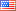Ask Your Question

# Revision history [back]

### How do I get the output of this text in latex with sagetex?

Using Sage\TeX, one can use Sage to compute things and put them into your \LaTeX{} document. For example, there are $\sage{number_of_partitions(1269)}$ integer partitions of $1269$. You don't need to compute the number yourself, or even cut and paste it from somewhere.

Here's some Sage code:

\begin{sageblock} f(x) = exp(x) * sin(2*x) \end{sageblock}

The second derivative of $f$ is

[ \frac{\mathrm{d}^{2}}{\mathrm{d}x^{2}} \sage{f(x)} = \sage{diff(f, x, 2)(x)}. ]

Here's a plot of $f$ from $-1$ to $1$:

\sageplot{plot(f, -1, 1)}

\end{document}2 NoneJohn Palmieri6339 ●18 ●56 ●141 http://www.math.washin...

### How do I get the output of this text in latex with sagetex?

Using Sage\TeX, one can use Sage to compute things and put them into your \LaTeX{} document. For example, there are $\sage{number_of_partitions(1269)}$ $\sage{number_of_partitions(1269)}$ integer partitions of $1269$. You don't need to compute the number yourself, or even cut and paste it from somewhere.

Here's some Sage code:

\begin{sageblock} f(x) = exp(x) * sin(2*x) \end{sageblock}

The second derivative of $f$ is

[ \frac{\mathrm{d}^{2}}{\mathrm{d}x^{2}} \sage{f(x)} = \sage{diff(f, x, 2)(x)}. ]

Here's a plot of $f$ from $-1$ to $1$:

\sageplot{plot(f, -1, 1)}

\end{document}